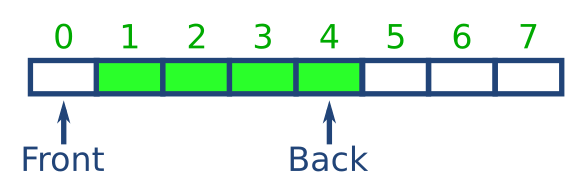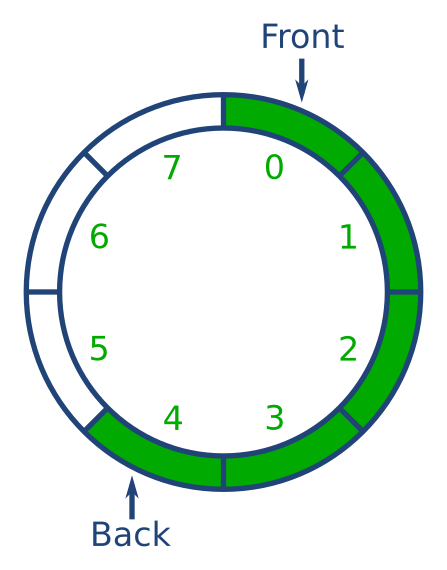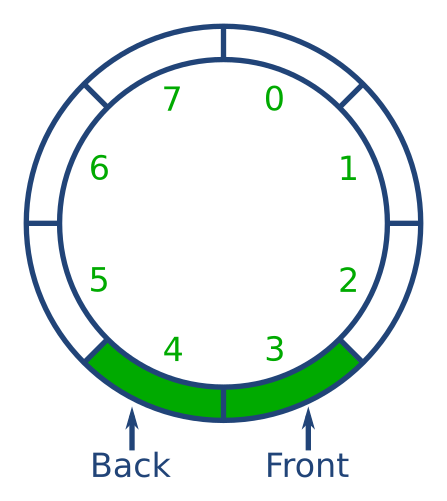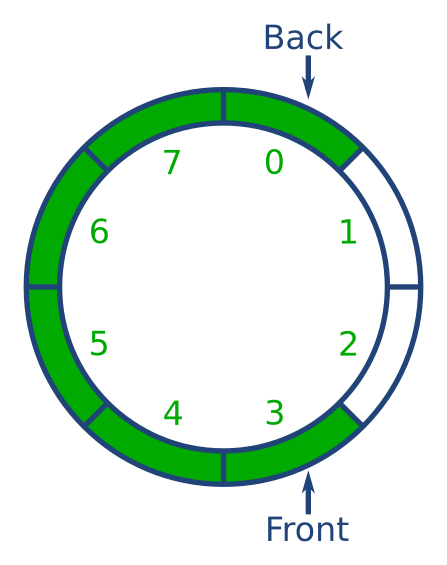# Circular queues

Martin McBride, 2018-05-05
Tags queue circular queue
Categories data structures

A queue can be created from a static array (sometimes called a buffer). Since an array is a static data structure, the queue created will also be static (meaning that the size of the queue cannot be changed after it is created).

Implementing a queue based on an array is inefficient, but a simple trick of using a circular buffer can make a big improvement.

## Using a normal array

Here is a queue based on a static array of 8 elements. The queue can hold up to 8 items, after which it becomes full. Here the queue has 5 items, shown in green:Now look what happens when we take an element off the queue:The remaining elements are in the wrong place in the aray. Since we are using an array rather than a list, we cannot simply remove element 0. We need to shift all the elements down to remove the gap. Moving elements around like this takes time so it slows the queue operations down.

## Using a circular buffer

A circular buffer has a fixed size, like a normal array. The difference is that when the index of the buffer reaches the maximum values, it wraps back round to 0.

Here is a circular buffer of length 8. It has 5 elements in it, marked in green, with the other 3 elements unused:Now we will remove some elements from the front of the queue. However, each time we remove an elements, we don't need to shift the elements along the buffer. We just move the Front index to point at the new start of the queue. Here is what it looks like after removing 3 items from the front of the queue:Next we will add some elements to the back of the queue. To do this, we just add an element and move the Back index to point at the new back of the queue.

When the back index reaches 7, the next time we increment it we set it to 0 (rather than 8), and start reusing the empty elements at the start of the array. Here we have added 4 new elements:## Handling the indices

Keeping track of the Front and Back indices might seem complicated, but in fact it is easy. Each time to add 1 to an index, you use modulo arithmetic:

i = (i + 1) % 8


Here we are using modulo 8 because the buffer is 8 long - use the value that matches your buffer. The modulo (%) operator returns the remainder when the value is divided by, in this case, 8.

So if the index is at 7 and we add 1 to it, giving 8, the result of the modulo is 0 (because 8 divided by 8 is 1 remainder 0). Provided we use modulo arithmetic, we can keep adding 1 to the indices and they will loop round and round.

## Python implementation

Here is a circular queue in Python. It is for illustration only, you should use the collections.deque implementation if you want an efficient queue in Python.

queue = *8
front = 0
back = 0
size = 0

def isEmpty():
global size
return size 0

def isFull():
global size
return size 8

def enQueue(v):
global size
global back
if isFull():
print('Error queue is full')
else:
queue[back] = v
back = (back + 1) % 8
size += 1

def deQueue():
global size
global front
if isEmpty():
print('Error queue is empty')
else:
v = queue[front]
front = (front + 1) % 8
size -= 1
return v


Here we declare several global variables:

• queue the array to hold the queue elements
• front the index of the front element
• back the index of the back element
• size the number of valid items in the queue

The queue is empty if size is 0. The queue is full if size is 8.

We enQueue an item by placing it in the array at the back index. We increment the back index (modulo 8) and increment the size.

We deQueue an item by reading it from the array at the front index. We increment the front index (modulo 8) and decrement the size.